# KSEEB Solutions for Class 9 Maths Chapter 1 Number Systems Ex 1.5

In this chapter, we provide KSEEB SSLC Class 9 Maths Chapter 1 Number Systems Ex 1.5 for English medium students, Which will very helpful for every student in their exams. Students can download the latest KSEEB SSLC Class 9 Maths Chapter 1 Number Systems Ex 1.5 pdf, free KSEEB SSLC Class 9 Maths Chapter 1 Number Systems Ex 1.5 pdf download. Now you will get step by step solution to each question.

## Karnataka Board Class 9 Maths Chapter 1 Number Systems Ex 1.5

Question 1.
Classify the following numbers as rational or irrational.
i) 2−5–√
ii) (3+23−−√)−23−−√
iii) 27√77√
iv) 12√
v) 2π
i) 2−5–√ = 2 – 2.2360679……….. = -0.2360679
This is a non-terminating, non-recurring decimal.
∴ This is an irrational number.

ii) (3+23−−√)−23−−√
= 3+23−−√−23−−√
= 3
⇒ 31. This can be written in the form of pq.
∴ This is a rationl number.

iii) 27√77√=27√7√=2⇒21
This can be written in the form of pq
∴ This is a rational number.

iv) 12√
12√×2√2√=2√2=1.41422
= 0.707106…………
This is a non-terminating, non-recurring decimal.
∴ This is an irrational number.

v) 2π
= 2 × 3.1415…..
= 6.2830…….
This is a non-terminating, non-recurring decimal.
∴ This is an irrational number.

Question 2.
Simplify each of the following expressions:
i) (3+3–√)(2+2–√)
ii) (3+3–√)(3−3–√)
iii) (5–√+2–√)2
iv) (5–√−2–√)(5–√+2–√)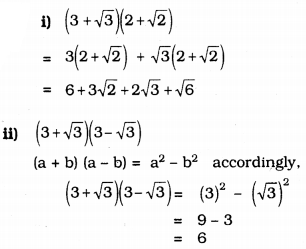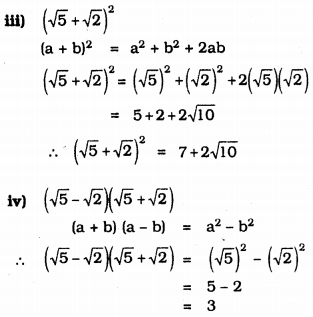Question 3.
Recall, 2π is defined as the ratio of the circumference (say c) of a circle to its diameter (say d). That is, π=cd. This seems to contradict the fact that n is irrational. How will you resolve this contradiction?
There is no contradiction. When we measure a length with a scale or any other device we get the quotient.
Therefore we cannot judge whether c is a rational number of d is an irrational number.
∴ Value of cd is irrational number.
∴ The value of π is also an irrational number.

Question 4.
Represent 9.3−−−√ on the number line.
Construction: Mark the distance 9.5 units from a fixed point O such that OB = 9.3 units. Mark midpoint D of OC. Draw a semicircle with centre D. Draw a line perpendicular to OC passing through E and intersecting the semicircle at E. Draw an arc BE which intersect at F. Now, BF = 9.3−−−√.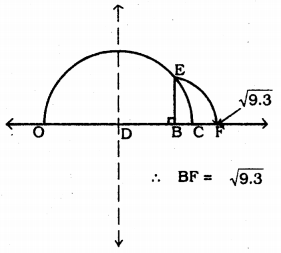Question 5.
Rationalise the denominators of the following :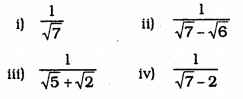i) 17√
Denominator’s factor is 7–√ Mulitplying Numerator and denominator by 7–√.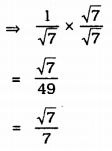ii) 17√−6√
Denominator’s factor is 7–√+6–√
Multiplying numerator and denominator by 7–√+6–√,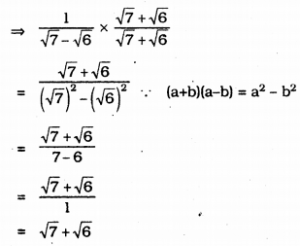iii) 15√+2√
Denominator’s factor is 5–√−2–√
Multiplying numerator and denominator by 5–√−2–√.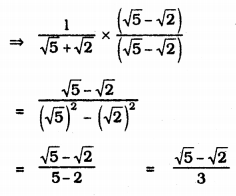iv) 17√−2
Denominator’s factor is 7–√+2
Multiplying numerator and denominator by 7–√+2.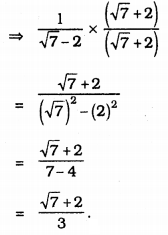All Chapter KSEEB Solutions For Class 9 Maths

—————————————————————————–

All Subject KSEEB Solutions For Class 9

*************************************************

I think you got complete solutions for this chapter. If You have any queries regarding this chapter, please comment on the below section our subject teacher will answer you. We tried our best to give complete solutions so you got good marks in your exam.

If these solutions have helped you, you can also share kseebsolutionsfor.com to your friends.

Best of Luck!!